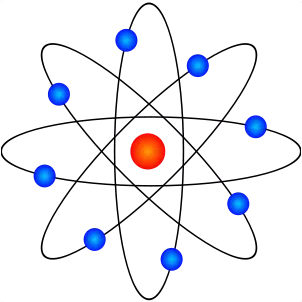# Top 20 Physics Interview Questions & Answers

1)      Explain what is Quantum Physics?

The understanding of behaviour of matter and energy at the molecular, nuclear, atomic and even microscopic levels is referred as Quantum physics

2)      Explain what is Quantum entanglement?

Quantum entanglement is one of the central principle of quantum physics, which means multiple particles are linked together in a way that the measurement of one particle quantum state determines the possible quantum of the other particles

3)      Explain what is shearing stress?

Shearing stress is the ratio of the tangential force F to the area of the face BCGH over which it is applied.  The ratio shearing stress is divided by shearing strain is the shear module or co-efficient of rigidity, n

Shearing stress        =   const

Shearing strain

4)      Mention what is the speed of light in space?

In the space, light travels at a speed of 186,282 miles per second and sunlight takes about 8 min and 19 sec to reach the surface of the earth.5)      What are the properties of fourth matter Plasma?

After Solid, liquid and gas there is one more matter that exists known as Plasma.  Properties of Plasma are

• Plasma has neither a definite shape nor a definite volume
• Plasma often seen in ionized gases, and heating produces it and ionizing a gas
• Free electrical charges which are not bound to atoms or ions can cause plasma to be electrically conductive
• Some of the examples of plasma are lightning, stars, inside fluorescent lights and neon signs

6)      Define what is Thermal Conductivity?

The property of the material that relates to its ability to conduct heat is referred as Thermal Conductivity.

7)      Explain what is Parallax and Distance Measurement?

To measure the distance to nearby stars, astronomers use an effect called Parallax.  Parallax is the apparent displacement of an object because of a change in observer’s point of view, for instance, when we look at object with one eye shut and then doing the same with other eye, there is a difference in the position of the object this is known as Parallax.

To measure the star position astronomer uses this technique. Once the star position is located after six month they will again calculate the apparent change in position.

8)      Mention what is the unit to measure the heat resistance?

Ohm is the unit to measure the heat resistance.

9)      What is the instrument used to know the scattering of light by particles suspended in a liquid?

Nephelometer is the instrument used to measure the scattering of light by particles suspended in a liquid

10)   Explain what is dyne?

Dyne is a unit of force or also referred as C-G-S (centimetre – gram –-second).  It means that when a force is applied to mass of 1 gram, it gives acceleration of 1 centimetre per second.

11)   Explain what is Wave-Particle duality?

When matter and light exhibits properties of both waves and particles, it is referred as Wave-Particle duality.  For instance, Light can behave like wave when it shines through narrow slits while, when exposed to some metal surface it will spray electrons acting as a particle. So under different conditions it will act.

12)   Explain what is Quantum tunnelling?

Quantum tunnelling is the process where the particle passes through an obstruction or barrier to reach at another end.  It is referred as tunnelling as the particle as “dug” out the way through the potential barrier.

13)   Explain what is Uncertainty principle?

Uncertainty principle tells that the momentum and position of a particle cannot be measured precisely.

14)   Explain what is dark matter?

Dark matter is an invisible matter in the space that can hold the stars into the galaxy.  They have no effect of electromagnetic force on it, which means it does absorb, reflect or emit light that makes them practically invisible.

15)   Explain what is a photon under the photon theory of light?

A discrete bundle of electromagnetic light or energy, which always remains in motion is referred as photon.

16)   Mention what are the properties of Photon?

• It moves at a constant velocity
• It has zero mass and rest energy
• When exposed (absorbed/emitted) to radiation it can be destroyed or created
• With the electron and another particle it will show particle like interaction
• It carries energy and momentum

17)   Explain what is Pascal law?

A Pascal law states that when you apply force at one point on liquid it will transmit equal force from one location to another within the liquid.

18)   Explain what is Neutrino?

Neutrino is a small, tiny elementary particle which carries no electrical charge which means it is not affected by electro-magnetic forces, and travels almost the speed of light and passes through ordinary matter without making any interaction.

19)   Mention the factors on which the velocity of sound depends?

Velocity of sound depends on velocity and density of the medium on which it travels.  It varies directly as the square root of elasticity and inversely as the square root of the density.

20)   Define term “Convection”?

Convection is the process of transferring heat by movement of heated fluid such as water or air.  In this process, the heated fluid expands, and gravity pulls the denser masses under them thus forcing them into motion. One good example is a draft of lamps and stoves.

Bonus Question

21)   Explain the term angular acceleration?

Angular acceleration is the rate of change of angular velocity or speed of a body moving along a circular path.

4 replies

1.arun kumar says:

Sir , I’m bsc ( physics) student . I want to prepare the professor.
How do?

2.Uma says:

Thank you sir.

3.4.